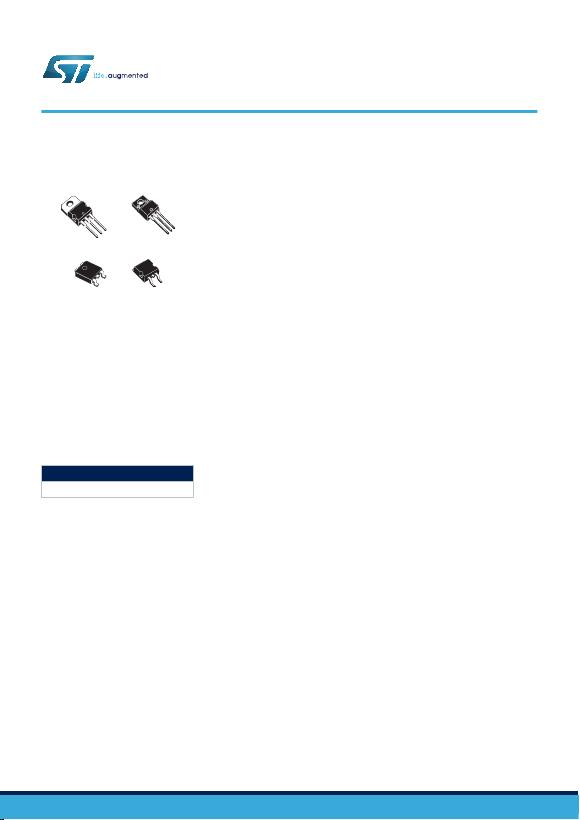TO-220FPTO-220
D²PAK
DPAK
Features
Output current up to 1.5 A
Output voltages of 5; 6; 8; 8.5; 9; 12; 15; 18; 24 V
Thermal overload protection
Short circuit protection
Output transition SOA protection
2 % output voltage tolerance (A version)
Guaranteed in extended temperature range (A version)
Description
The L78 series of three-terminal positive regulators is available in TO-220,
TO-220FP, D²PAK and DPAK packages and several fixed output voltages, making it
useful in a wide range of applications.
These regulators can provide local on-card regulation, eliminating the distribution
problems associated with single point regulation. Each type embeds internal current
limiting, thermal shut-down and safe area protection, making it essentially
indestructible. If adequate heat sinking is provided, they can deliver over 1 A output
current. Although designed primarily as fixed voltage regulators, these devices can
be used with external components to obtain adjustable voltage and currents.
Maturity status link
L78
Positive voltage regulator ICs
L78
Datasheet
DS0422 - Rev 36 - September 2018
For further information contact your local STMicroelectronics sales office.
www.st.com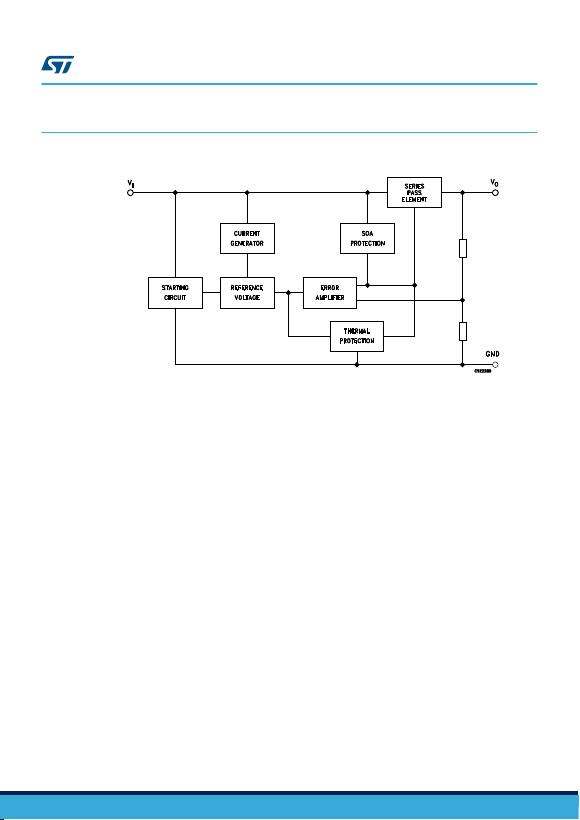1Diagram
Figure 2. Block diagram
GAMG220920161000MT
L78
Diagram
DS0422 - Rev 36 page 2/55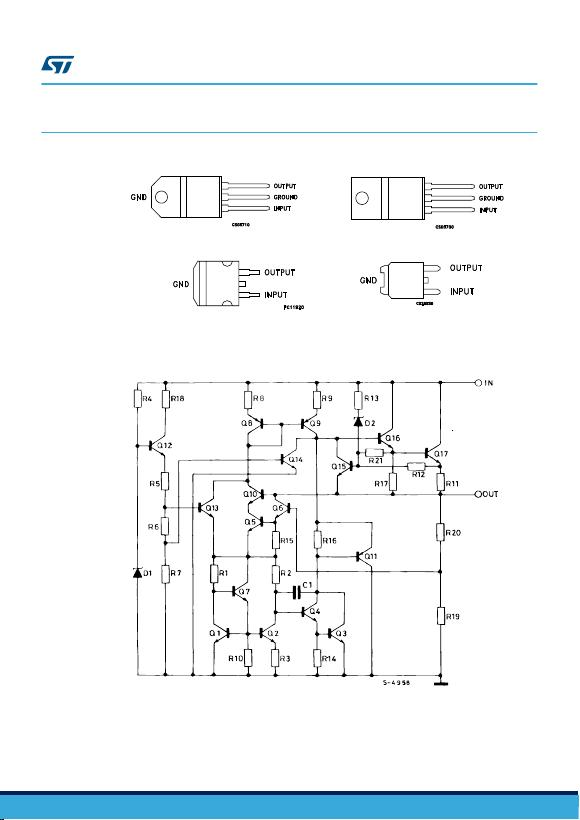2Pin configuration
Figure 3. Pin connections (top view)
TO-220FP
TO-220
D²PAK DPAK
GAMG220920161001MT
Figure 4. Schematic diagram
GAMG220920161002MT
L78
Pin configuration
DS0422 - Rev 36 page 3/55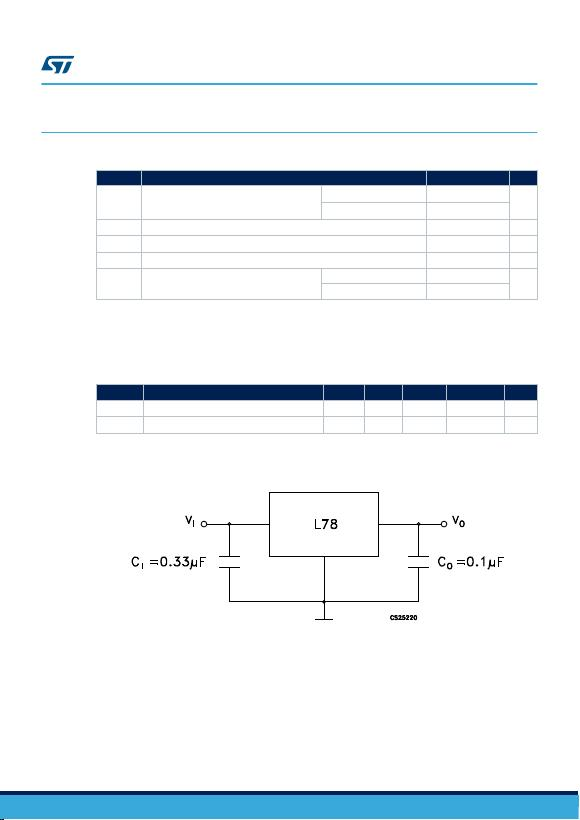3Maximum ratings
Table 1. Absolute maximum ratings
Symbol Parameter Value Unit
VIDC input voltage
for VO= 5 to 18 V 35
V
for VO= 20, 24 V 40
IOOutput current Internally limited
PDPower dissipation Internally limited
TSTG Storage temperature range -65 to 150 °C
TOP Operating junction temperature range
for L78xxC, L78xxAC 0 to 125
°C
for L78xxAB -40 to 125
Note: Absolute maximum ratings are those values beyond which damage to the device may occur. Functional
operation under these condition is not implied.
Table 2. Thermal data
Symbol Parameter D²PAK DPAK TO-220 TO-220FP Unit
RthJC Thermal resistance junction-case 3 8 5 5 °C/W
RthJA Thermal resistance junction-ambient 62.5 100 50 60 °C/W
Figure 5. Application circuits
GAMG220920161003MT
L78
Maximum ratings
DS0422 - Rev 36 page 4/55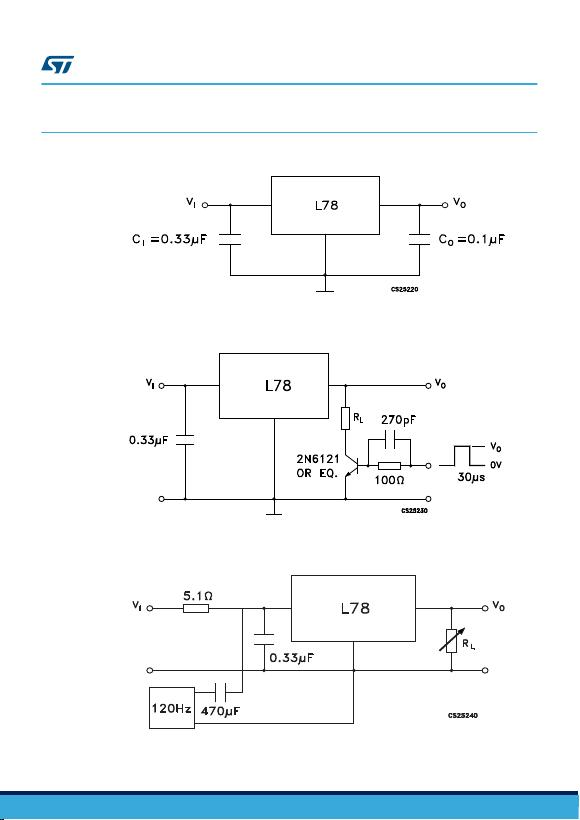4Test circuits
Figure 6. DC parameter
GAMG220920161004MT
Figure 7. Load regulation
GAMG220920161005MT
Figure 8. Ripple rejection
GAMG220920161006MT
L78
Test circuits
DS0422 - Rev 36 page 5/55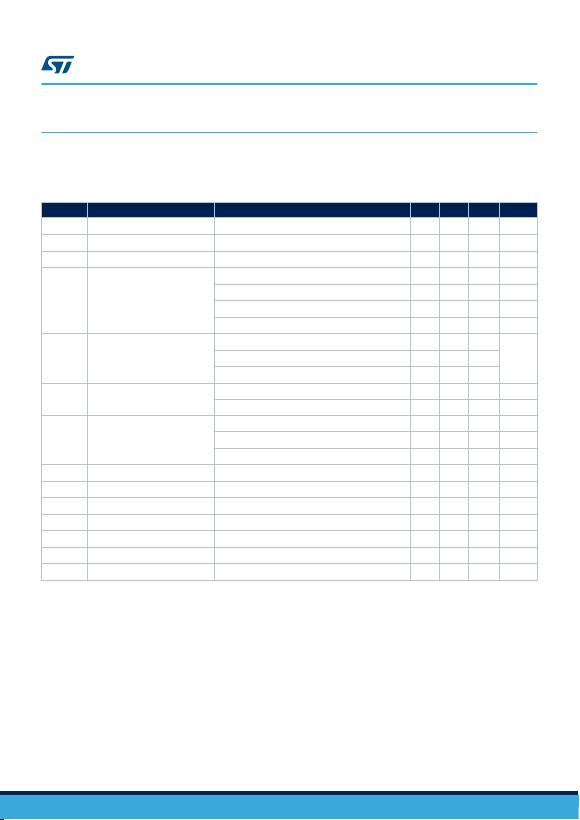5Electrical characteristics
VI = 10 V, IO = 1 A, TJ = 0 to 125 °C (L7805AC), TJ = -40 to 125 °C (L7805AB), unless otherwise specified.
Table 3. Electrical characteristics of L7805A
Symbol Parameter Test conditions Min. Typ. Max. Unit
VOOutput voltage TJ = 25 °C 4.9 5 5.1 V
VOOutput voltage IO = 5 mA to 1 A, VI = 7.5 to 18 V 4.8 5 5.2 V
VOOutput voltage IO = 1 A, VI = 18 to 20 V, TJ = 25 °C 4.8 5 5.2 V
∆VO (1) Line regulation
VI = 7.5 to 25 V, IO = 500 mA, TJ = 25 °C 7 50 mV
VI = 8 to 12 V 10 50 mV
VI = 8 to 12 V, TJ = 25 °C 2 25 mV
VI = 7.3 to 20 V, TJ = 25 °C 7 50 mV
∆VO (1) Load regulation
IO = 5 mA to 1 A 25 100
mV
IO = 5 mA to 1.5 A, TJ = 25 °C 30 100
IO = 250 to 750 mA 8 50
IqQuiescent current
TJ = 25 °C 4.3 6 mA
6 mA
∆IqQuiescent current change
VI = 8 to 23 V, IO = 500 mA 0.8 mA
VI = 7.5 to 20 V, TJ = 25 °C 0.8 mA
IO = 5 mA to 1 A 0.5 mA
SVR Supply voltage rejection VI = 8 to 18 V, f = 120 Hz, IO = 500 mA 68 dB
VdDropout voltage IO = 1 A, TJ = 25 °C 2 V
eN Output noise voltage TA = 25 °C, B =10 Hz to 100 kHz 10 µV/VO
ROOutput resistance f = 1 kHz 17 mΩ
Isc Short circuit current VI = 35 V, TA = 25 °C 0.2 A
Iscp Short circuit peak current TJ = 25 °C 2.2 A
∆VO/∆T Output voltage drift -1.1 mV/°C
1. Load and line regulation are specified at constant junction temperature. Changes in VO due to heating
effects must be taken into account separately. Pulse testing with low duty cycle is used.
Note: Minimum load current for regulation is 5 mA.
L78
Electrical characteristics
DS0422 - Rev 36 page 6/55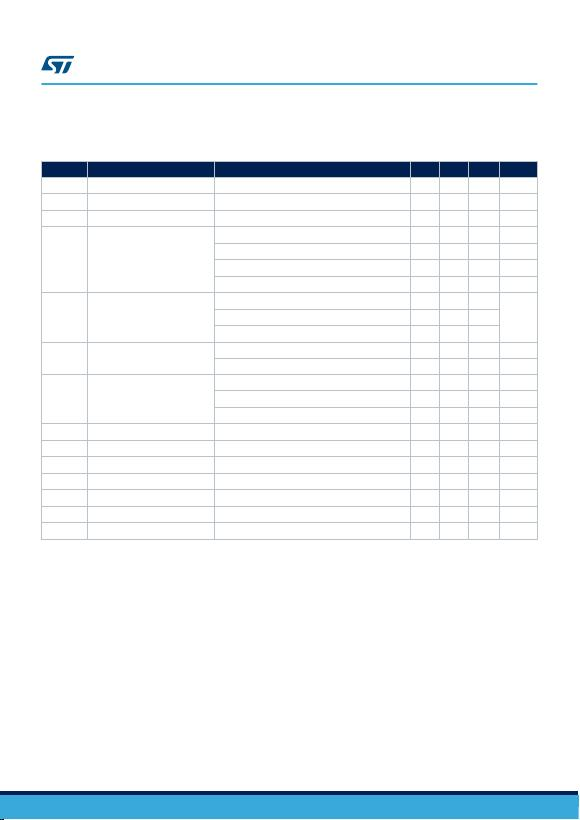VI = 11 V, IO = 1 A, TJ = 0 to 125 °C (L7806AC), TJ = -40 to 125 °C (L7806AB), unless otherwise specified.
Table 4. Electrical characteristics of L7806A
Symbol Parameter Test conditions Min. Typ. Max. Unit
VOOutput voltage TJ = 25 °C 5.88 6 6.12 V
VOOutput voltage IO = 5 mA to 1 A, VI = 8.6 to 19 V 5.76 6 6.24 V
VOOutput voltage IO = 1 A, VI = 19 to 21 V, TJ = 25 °C 5.76 6 6.24 V
∆VO (1) Line regulation
VI = 8.6 to 25 V, IO = 500 mA, TJ = 25 °C 9 60 mV
VI = 9 to 13 V 11 60 mV
VI = 9 to 13 V, TJ = 25 °C 3 30 mV
VI = 8.3 to 21 V, TJ = 25 °C 9 60 mV
∆VO (1) Load regulation
IO = 5 mA to 1 A 25 100
mV
IO = 5 mA to 1.5 A, TJ = 25 °C 30 100
IO = 250 to 750 mA 10 50
IqQuiescent current
TJ = 25° C 4.3 6 mA
6 mA
∆IqQuiescent current change
VI = 9 to 24 V, IO = 500 mA 0.8 mA
VI = 8.6 to 21 V, TJ = 25 °C 0.8 mA
IO = 5 mA to 1 A 0.5 mA
SVR Supply voltage rejection VI = 9 to 19 V, f = 120 Hz, IO = 500 mA 65 dB
VdDropout voltage IO = 1 A, TJ = 25 °C 2 V
eN Output noise voltage TA = 25 °C, B =10 Hz to 100 kHz 10 µV/VO
ROOutput resistance f = 1 kHz 17 mΩ
Isc Short circuit current VI = 35 V, TA = 25 °C 0.2 A
Iscp Short circuit peak current TJ = 25 °C 2.2 A
∆VO/∆T Output voltage drift -0.8 mV/°C
1. Load and line regulation are specified at constant junction temperature. Changes in VO due to heating
effects must be taken into account separately. Pulse testing with low duty cycle is used.
Note: Minimum load current for regulation is 5 mA.
L78
Electrical characteristics
DS0422 - Rev 36 page 7/55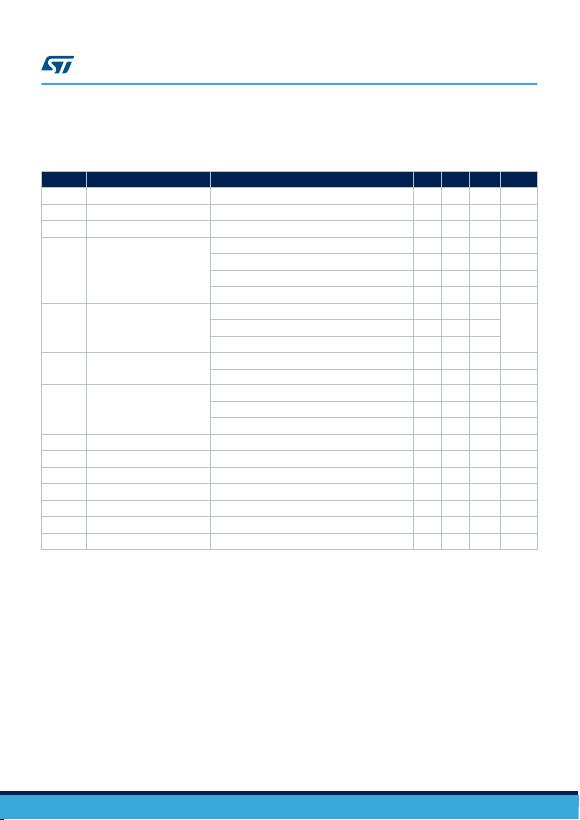VI = 14 V, IO = 1 A, TJ = 0 to 125 °C (L7808AC), TJ = -40 to 125 °C (L7808AB), unless otherwise specified.
Table 5. Electrical characteristics of L7808A
Symbol Parameter Test conditions Min. Typ. Max. Unit
VOOutput voltage TJ = 25 °C 7.84 8 8.16 V
VOOutput voltage IO = 5 mA to 1 A, VI = 10.6 to 21 V 7.7 8 8.3 V
VOOutput voltage IO = 1 A, VI = 21 to 23 V, TJ = 25 °C 7.7 8 8.3 V
∆VO (1) Line regulation
VI = 10.6 to 25 V, IO = 500 mA, TJ = 25 °C 12 80 mV
VI = 11 to 17 V 15 80 mV
VI = 11 to 17 V, TJ = 25 °C 5 40 mV
VI = 10.4 to 23 V, TJ = 25 °C 12 80 mV
∆VO (1) Load regulation
IO = 5 mA to 1 A 25 100
mV
IO = 5 mA to 1.5 A, TJ = 25 °C 30 100
IO = 250 to 750 mA 10 50
IqQuiescent current
TJ = 25 °C 4.3 6 mA
6 mA
∆IqQuiescent current change
VI = 11 to 23 V, IO = 500 mA 0.8 mA
VI = 10.6 to 23 V, TJ = 25 °C 0.8 mA
IO = 5 mA to 1 A 0.5 mA
SVR Supply voltage rejection VI = 11.5 to 21.5 V, f = 120 Hz, IO = 500 mA 62 dB
VdDropout voltage IO = 1 A, TJ = 25 °C 2 V
eN Output noise voltage TA = 25 °C, B =10 Hz to 100 kHz 10 µV/VO
ROOutput resistance f = 1 kHz 18 mΩ
Isc Short circuit current VI = 35 V, TA = 25 °C 0.2 A
Iscp Short circuit peak current TJ = 25 °C 2.2 A
∆VO/∆T Output voltage drift -0.8 mV/°C
1. Load and line regulation are specified at constant junction temperature. Changes in VO due to heating
effects must be taken into account separately. Pulse testing with low duty cycle is used.
Note: Minimum load current for regulation is 5 mA.
L78
Electrical characteristics
DS0422 - Rev 36 page 8/55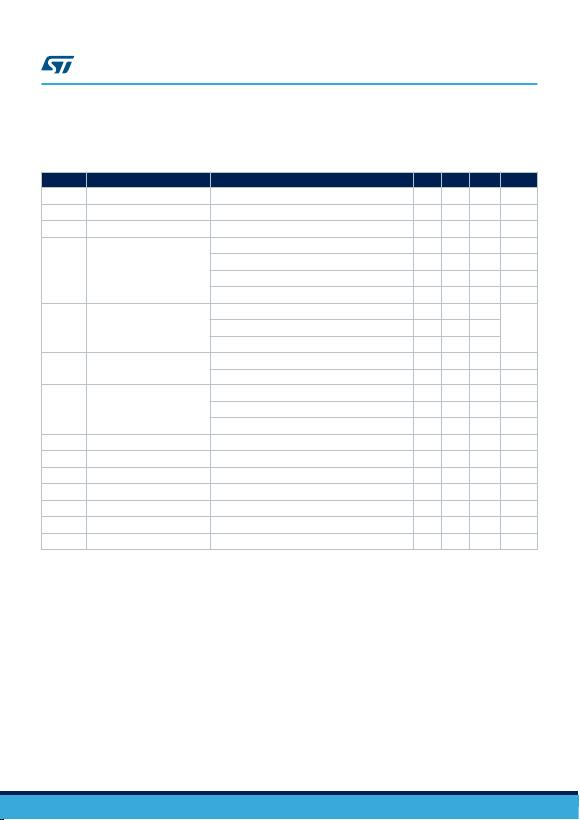VI = 15 V, IO = 1 A, TJ = 0 to 125 °C (L7809AC), TJ = -40 to 125 °C (L7809AB), unless otherwise
specified(Minimum load current for regulation is 5 mA.)
Table 6. Electrical characteristics of L7809A
Symbol Parameter Test conditions Min. Typ. Max. Unit
VOOutput voltage TJ = 25 °C 8.82 9 9.18 V
VOOutput voltage IO = 5 mA to 1 A, VI = 10.6 to 22 V 8.65 9 9.35 V
VOOutput voltage IO = 1 A, VI = 22 to 24 V, TJ = 25 °C 8.65 9 9.35 V
∆VO (1) Line regulation
VI = 10.6 to 25 V, IO = 500 mA, TJ = 25 °C 12 90 mV
VI = 11 to 17 V 15 90 mV
VI = 11 to 17 V, TJ = 25 °C 5 45 mV
VI = 11.4 to 23 V, TJ = 25 °C 12 90 mV
∆VO (1) Load regulation
IO = 5 mA to 1 A 25 100
mV
IO = 5 mA to 1.5 A, TJ = 25 °C 30 100
IO = 250 to 750 mA 10 50
IqQuiescent current
TJ = 25 °C 4.3 6 mA
6 mA
∆IqQuiescent current change
VI = 11 to 25 V, IO = 500 mA 0.8 mA
VI = 10.6 to 23 V, TJ = 25 °C 0.8 mA
IO = 5 mA to 1 A 0.5 mA
SVR Supply voltage rejection VI = 11.5 to 21.5 V, f = 120 Hz, IO = 500 mA 61 dB
VdDropout voltage IO = 1 A, TJ = 25 °C 2 V
eN Output noise voltage TA = 25 °C, B =10 Hz to 100 kHz 10 µV/VO
ROOutput resistance f = 1 kHz 18 mΩ
Isc Short circuit current VI = 35 V, TA = 25 °C 0.2 A
Iscp Short circuit peak current TJ = 25 °C 2.2 A
∆VO/∆T Output voltage drift -0.8 mV/°C
1. Load and line regulation are specified at constant junction temperature. Changes in VO due to heating
effects must be taken into account separately. Pulse testing with low duty cycle is used.
Note: Minimum load current for regulation is 5 mA.
L78
Electrical characteristics
DS0422 - Rev 36 page 9/55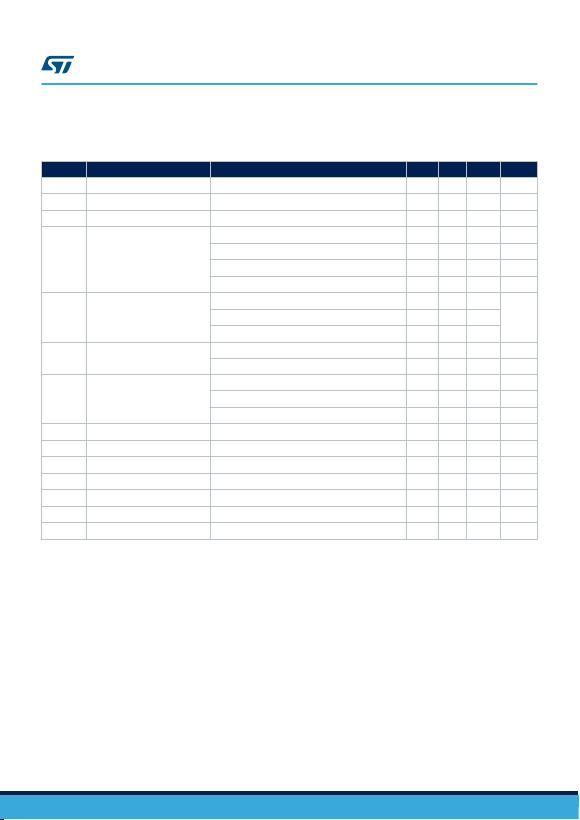VI = 19 V, IO = 1 A, TJ = 0 to 125 °C (L7812AC), TJ = -40 to 125 °C (L7812AB), unless otherwise specified.
Table 7. Electrical characteristics of L7812A
Symbol Parameter Test conditions Min. Typ. Max. Unit
VOOutput voltage TJ = 25 °C 11.75 12 12.25 V
VOOutput voltage IO = 5 mA to 1 A, VI = 14.8 to 25 V 11.5 12 12.5 V
VOOutput voltage IO = 1 A, VI = 25 to 27 V, TJ = 25 °C 11.5 12 12.5 V
∆VO (1) Line regulation
VI = 14.8 to 30 V, IO = 500 mA, TJ = 25 °C 13 120 mV
VI = 16 to 12 V 16 120 mV
VI = 16 to 12 V, TJ = 25 °C 6 60 mV
VI = 14.5 to 27 V, TJ = 25 °C 13 120 mV
∆VO (1) Load regulation
IO = 5 mA to 1 A 25 100
mV
IO = 5 mA to 1.5 A, TJ = 25 °C 30 100
IO = 250 to 750 mA 10 50
IqQuiescent current
TJ = 25 °C 4.4 6 mA
6 mA
DIqQuiescent current change
VI = 15 to 30 V, IO = 500 mA 0.8 mA
VI = 14.8 to 27 V, TJ = 25 °C 0.8 mA
IO = 5 mA to 1 A 0.5 mA
SVR Supply voltage rejection VI = 15 to 25 V, f = 120 Hz, IO = 500 mA 60 dB
VdDropout voltage IO = 1 A, TJ = 25 °C 2 V
eN Output noise voltage TA = 25 °C, B = 10 Hz to 100 kHz 10 µV/VO
ROOutput resistance f = 1 kHz 18 mΩ
Isc Short circuit current VI = 35 V, TA = 25 °C 0.2 A
Iscp Short circuit peak current TJ = 25 °C 2.2 A
∆VO/∆T Output voltage drift -1 mV/°C
1. Load and line regulation are specified at constant junction temperature. Changes in VO due to heating
effects must be taken into account separately. Pulse testing with low duty cycle is used.
Note: Minimum load current for regulation is 5 mA.
L78
Electrical characteristics
DS0422 - Rev 36 page 10/55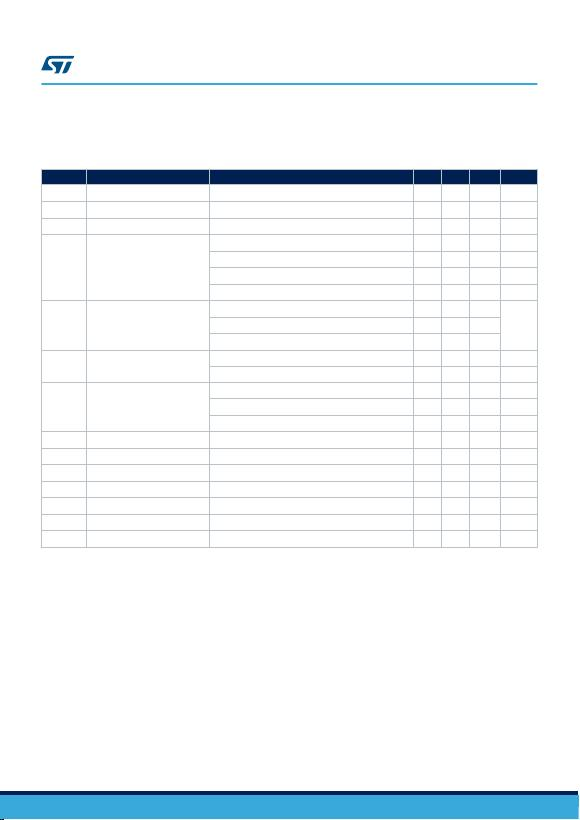VI = 23 V, IO = 1 A, TJ = 0 to 125 °C (L7815AC), TJ = -40 to 125 °C (L7815AB), unless otherwise specified.
Table 8. Electrical characteristics of L7815A
Symbol Parameter Test conditions Min. Typ. Max. Unit
VOOutput voltage TJ = 25 °C 14.7 15 15.3 V
VOOutput voltage IO = 5 mA to 1 A, VI = 17.9 to 28 V 14.4 15 15.6 V
VOOutput voltage IO = 1 A, VI = 28 to 30 V, TJ = 25 °C 14.4 15 15.6 V
∆VO (1) Line regulation
VI = 17.9 to 30 V, IO = 500 mA, TJ = 25 °C 13 150 mV
VI = 20 to 26 V 16 150 mV
VI = 20 to 26 V, TJ = 25 °C 6 75 mV
VI = 17.5 to 30 V, TJ = 25 °C 13 150 mV
∆VO (1) Load regulation
IO = 5 mA to 1 A 25 100
mV
IO = 5 mA to 1.5 A, TJ = 25 °C 30 100
IO = 250 to 750 mA 10 50
IqQuiescent current
TJ = 25 °C 4.4 6 mA
6 mA
∆IqQuiescent current change
VI = 17.5 to 30 V, IO = 500 mA 0.8 mA
VI = 17.5 to 30 V, TJ = 25 °C 0.8 mA
IO = 5 mA to 1 A 0.5 mA
SVR Supply voltage rejection VI = 18.5 to 28.5 V, f = 120 Hz, IO = 500 mA 58 dB
VdDropout voltage IO = 1 A, TJ = 25 °C 2 V
eN Output noise voltage TA = 25 °C, B = 10Hz to 100 kHz 10 µV/VO
ROOutput resistance f = 1 kHz 19 mΩ
Isc Short circuit current VI = 35 V, TA = 25 °C 0.2 A
Iscp Short circuit peak current TJ = 25 °C 2.2 A
∆VO/∆T Output voltage drift -1 mV/°C
1. Load and line regulation are specified at constant junction temperature. Changes in VO due to heating
effects must be taken into account separately. Pulse testing with low duty cycle is used.
Note: Minimum load current for regulation is 5 mA.
L78
Electrical characteristics
DS0422 - Rev 36 page 11/55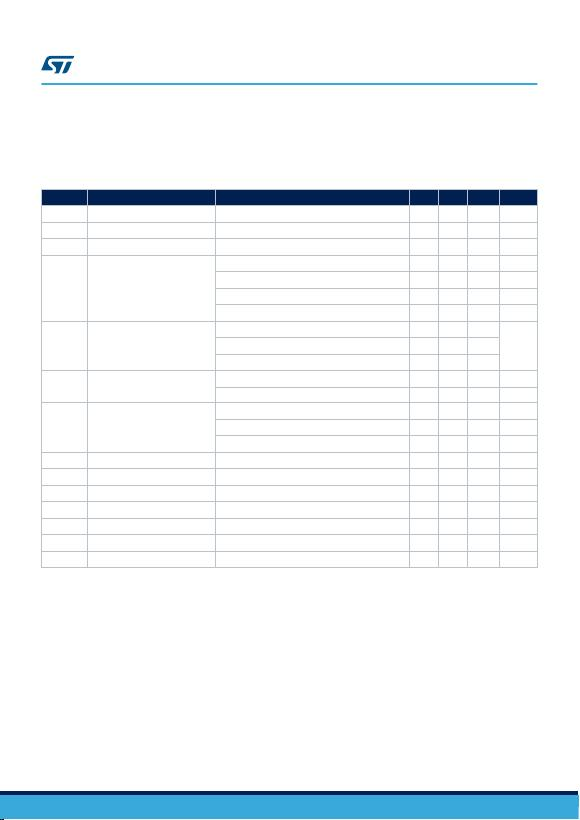VI = 33 V, IO = 1 A, TJ = 0 to 125 °C (L7824AC), TJ = -40 to 125 °C (L7824AB), unless otherwise specified.
Table 9. Electrical characteristics of L7824A
Symbol Parameter Test conditions Min. Typ. Max. Unit
VOOutput voltage TJ = 25 °C 23.5 24 24.5 V
VOOutput voltage IO = 5 mA to 1 A, VI = 27.3 to 37 V 23 24 25 V
VOOutput voltage IO = 1 A, VI = 37 to 38 V, TJ = 25 °C 23 24 25 V
∆VO (1) Line regulation
VI = 27 to 38 V, IO = 500 mA, TJ = 25 °C 31 240 mV
VI = 30 to 36 V 35 200 mV
VI = 30 to 36 V, TJ = 25 °C 14 120 mV
VI = 26.7 to 38 V, TJ = 25 °C 31 240 mV
∆VO (1) Load regulation
IO = 5 mA to 1 A 25 100
mV
IO = 5 mA to 1.5 A, TJ = 25 °C 30 100
IO = 250 to 750 mA 10 50
IqQuiescent current
TJ = 25 °C 4.6 6 mA
6 mA
∆IqQuiescent current change
VI = 27.3 to 38 V, IO = 500 mA 0.8 mA
VI = 27.3 to 38 V, TJ = 25 °C 0.8 mA
IO = 5 mA to 1 A 0.5 mA
SVR Supply voltage rejection VI = 28 to 38 V, f = 120 Hz, IO = 500 mA 54 dB
VdDropout voltage IO = 1 A, TJ = 25 °C 2 V
eN Output noise voltage TA = 25 °C, B = 10 Hz to 100 kHz 10 µV/VO
ROOutput resistance f = 1 kHz 20 m
Isc Short circuit current VI = 35 V, TA = 25 °C 0.2 A
Iscp Short circuit peak current TJ = 25 °C 2.2 A
∆VO/∆T Output voltage drift -1.5 mV/°C
1. Load and line regulation are specified at constant junction temperature. Changes in VO due to heating
effects must be taken into account separately. Pulse testing with low duty cycle is used.
Note: Minimum load current for regulation is 5 mA.
L78
Electrical characteristics
DS0422 - Rev 36 page 12/55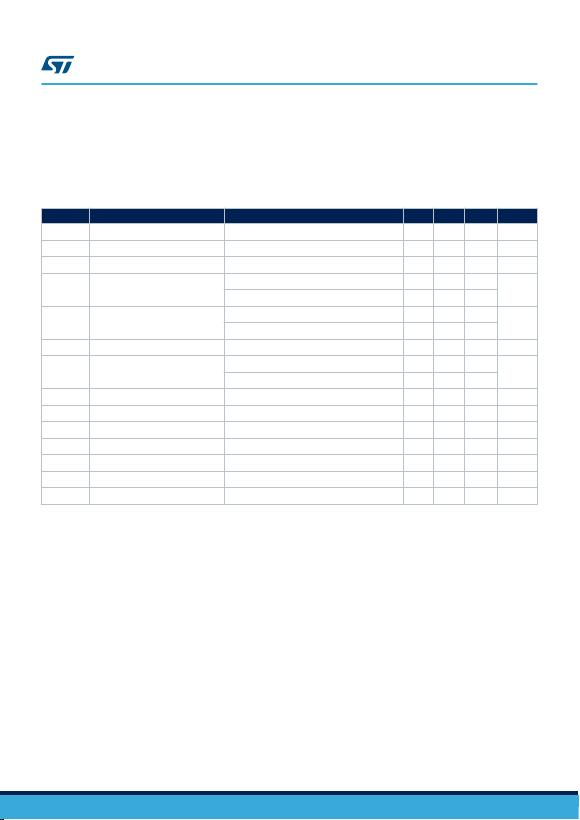Refer to the test circuits, TJ = 0 to 125 °C, VI = 10 V, IO = 500 mA, CI = 0.33 µF, CO = 0.1 µF unless otherwise
specified.
Table 10. Electrical characteristics of L7805C
Symbol Parameter Test conditions Min. Typ. Max. Unit
VOOutput voltage TJ = 25 °C 4.8 5 5.2 V
VOOutput voltage IO = 5 mA to 1 A, VI = 7 to 18 V 4.75 5 5.25 V
VOOutput voltage IO = 1 A, VI = 18 to 20V, TJ = 25 °C 4.75 5 5.25 V
∆VO (1) Line regulation
VI = 7 to 25 V, TJ = 25 °C 3 100
mV
VI = 8 to 12 V, TJ = 25 °C 1 50
∆VO (1) Load regulation
IO = 5 mA to 1.5 A, TJ = 25 °C 100
mV
IO = 250 to 750 mA, TJ = 25 °C 50
IdQuiescent current TJ = 25° C 8 mA
∆IdQuiescent current change
IO = 5 mA to 1 A 0.5
mA
VI = 7 to 23 V 0.8
∆VO/∆T Output voltage drift IO = 5 mA -1.1 mV/°C
eN Output noise voltage B = 10 Hz to 100 kHz, TJ = 25 °C 40 µV/VO
SVR Supply voltage rejection VI = 8 to 18 V, f = 120 Hz 62 dB
VdDropout voltage IO = 1 A, TJ = 25 °C 2 V
ROOutput resistance f = 1 kHz 17 mΩ
Isc Short circuit current VI = 35 V, TJ = 25 °C 0.75 A
Iscp Short circuit peak current TJ = 25 °C 2.2 A
1. Load and line regulation are specified at constant junction temperature. Changes in VO due to heating
effects must be taken into account separately. Pulse testing with low duty cycle is used.
Note: Minimum load current for regulation is 5 mA.
L78
Electrical characteristics
DS0422 - Rev 36 page 13/55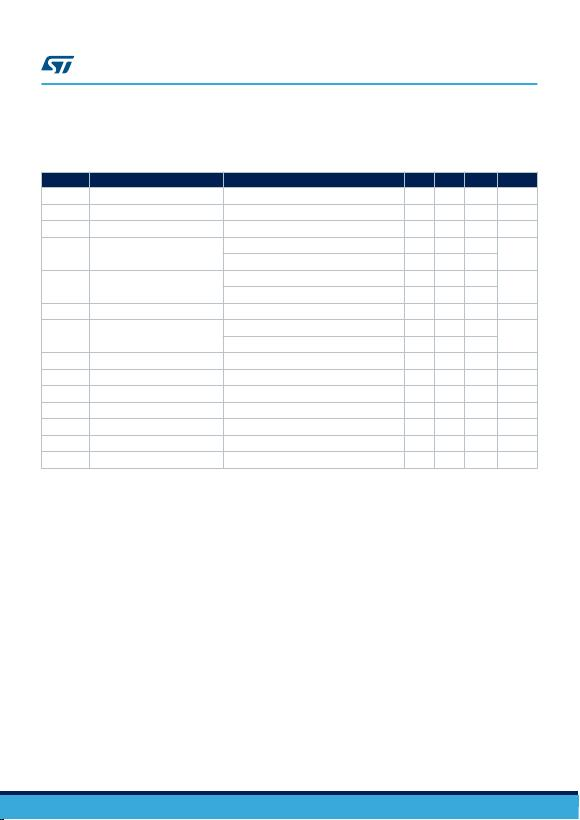Refer to the test circuits, TJ = 0 to 125 °C, VI = 11 V, IO = 500 mA, CI = 0.33 µF, CO = 0.1 µF unless otherwise
specified.
Table 11. Electrical characteristics of L7806C
Symbol Parameter Test conditions Min. Typ. Max. Unit
VOOutput voltage TJ = 25 °C 5.75 6 6.25 V
VOOutput voltage IO = 5 mA to 1 A, VI = 8 to 19 V 5.7 6 6.3 V
VOOutput voltage IO = 1 A, VI = 19 to 21 V, TJ = 25 °C 5.7 6 6.3 V
∆VO (1) Line regulation
VI = 8 to 25 V, TJ = 25 °C 120
mV
VI = 9 to 13 V, TJ = 25 °C 60
∆VO (1) Load regulation
IO = 5 mA to 1.5 A, TJ = 25 °C 120
mV
IO = 250 to 750 mA, TJ = 25 °C 60
IdQuiescent current TJ = 25 °C 8 mA
DIdQuiescent current change
IO = 5 mA to 1 A 0.5
mA
VI = 8 to 24 V 1.3
∆VO/∆T Output voltage drift IO = 5 mA -0.8 mV/°C
eN Output noise voltage B = 10 Hz to 100 kHz, TJ = 25 °C 45 µV/VO
SVR Supply voltage rejection VI = 9 to 19 V, f = 120 Hz 59 dB
VdDropout voltage IO = 1 A, TJ = 25 °C 2 V
ROOutput resistance f = 1 kHz 19 mΩ
Isc Short circuit current VI = 35 V, TJ = 25 °C 0.55 A
Iscp Short circuit peak current TJ = 25 °C 2.2 A
1. Load and line regulation are specified at constant junction temperature. Changes in VO due to heating
effects must be taken into account separately. Pulse testing with low duty cycle is used.
Note: Minimum load current for regulation is 5 mA.
L78
Electrical characteristics
DS0422 - Rev 36 page 14/55Refer to the test circuits, TJ = 0 to 125 °C, VI = 14 V, IO = 500 mA, CI = 0.33 µF, CO = 0.1 µF unless otherwise
specified.
Table 12. Electrical characteristics of L7808C
Symbol Parameter Test conditions Min. Typ. Max. Unit
VOOutput voltage TJ = 25 °C 7.7 8 8.3 V
VOOutput voltage IO = 5 mA to 1 A, VI = 10.5 to 21 V 7.6 8 8.4 V
VOOutput voltage IO = 1 A, VI = 21 to 25 V, TJ = 25 °C 7.6 8 8.4 V
∆VO (1) Line regulation
VI = 10.5 to 25 V, TJ = 25 °C 160
mV
VI = 11 to 17 V, TJ = 25 °C 80
∆VO (1) Load regulation
IO = 5 mA to 1.5 A, TJ = 25 °C 160
mV
IO = 250 to 750 mA, TJ = 25 °C 80
IdQuiescent current TJ = 25 °C 8 mA
∆IdQuiescent current change
IO = 5 mA to 1 A 0.5
mA
VI = 10.5 to 25 V 1
∆VO/∆T Output voltage drift IO = 5 mA -0.8 mV/°C
eN Output noise voltage B = 10 Hz to 100 kHz, TJ = 25 °C 52 µV/VO
SVR Supply voltage rejection VI = 11.5 to 21.5 V, f = 120 Hz 56 dB
VdDropout voltage IO = 1 A, TJ = 25 °C 2 V
ROOutput resistance f = 1 kHz 16 mΩ
Isc Short circuit current VI = 35 V, TJ = 25 °C 0.45 A
Iscp Short circuit peak current TJ = 25 °C 2.2 A
1. Load and line regulation are specified at constant junction temperature. Changes in VO due to heating
effects must be taken into account separately. Pulse testing with low duty cycle is used.
Note: Minimum load current for regulation is 5 mA.
L78
Electrical characteristics
DS0422 - Rev 36 page 15/55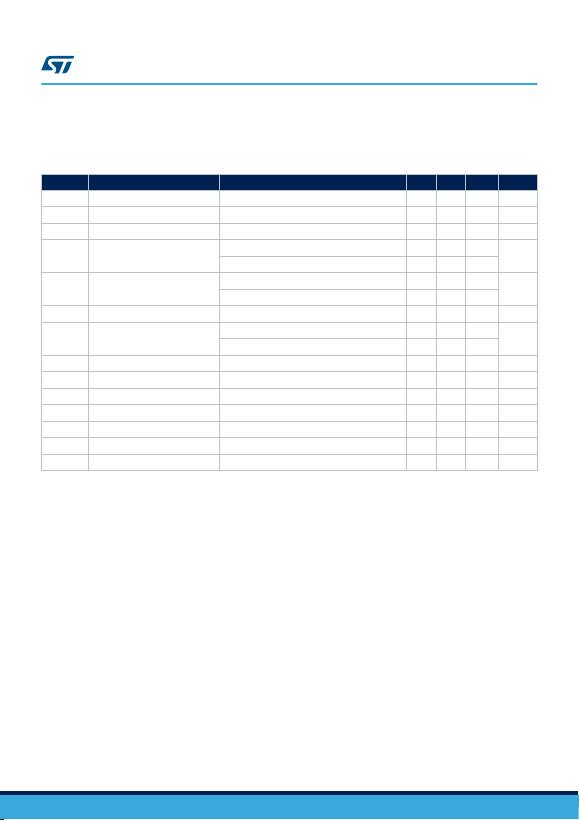Refer to the test circuits, TJ = 0 to 125 °C, VI = 14.5 V, IO = 500 mA, CI = 0.33 µF, CO = 0.1 µF unless otherwise
specified.
Table 13. Electrical characteristics of L7885C
Symbol Parameter Test conditions Min. Typ. Max. Unit
VOOutput voltage TJ = 25 °C 8.2 8.5 8.8 V
VOOutput voltage IO = 5 mA to 1 A, VI = 11 to 21.5 V 8.1 8.5 8.9 V
VOOutput voltage IO = 1 A, VI = 21.5 to 26 V, TJ = 25 °C 8.1 8.5 8.9 V
∆VO (1) Line regulation
VI = 11 to 27 V, TJ = 25 °C 160
mV
VI = 11.5 to 17.5 V, TJ = 25 °C 80
∆VO (1) Load regulation
IO = 5 mA to 1.5 A, TJ = 25 °C 160
mV
IO = 250 to 750 mA, TJ = 25 °C 80
IdQuiescent current TJ = 25 °C 8 mA
∆IdQuiescent current change
IO = 5 mA to 1 A 0.5
mA
VI = 11 to 26 V 1
∆VO/∆T Output voltage drift IO = 5 mA -0.8 mV/°C
eN Output noise voltage B = 10 Hz to 100 kHz, TJ = 25 °C 55 µV/VO
SVR Supply voltage rejection VI = 12 to 22 V, f = 120 Hz 56 dB
VdDropout voltage IO = 1 A, TJ = 25 °C 2 V
ROOutput resistance f = 1 kHz 16 mΩ
Isc Short circuit current VI = 35 V, TJ = 25 °C 0.45 A
Iscp Short circuit peak current TJ = 25 °C 2.2 A
1. Load and line regulation are specified at constant junction temperature. Changes in VO due to heating
effects must be taken into account separately. Pulse testing with low duty cycle is used.
Note: Minimum load current for regulation is 5 mA.
L78
Electrical characteristics
DS0422 - Rev 36 page 16/55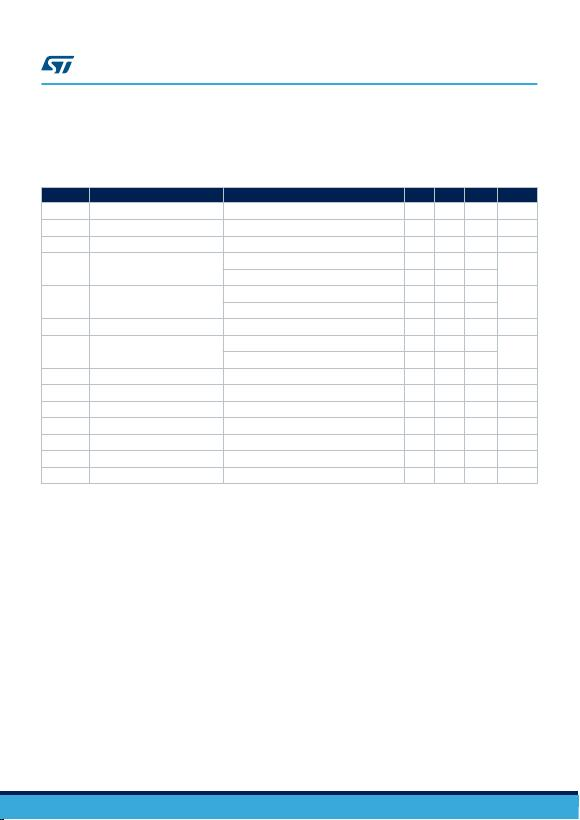Refer to the test circuits, TJ = 0 to 125 °C, VI = 15 V, IO = 500 mA, CI = 0.33 µF, CO = 0.1 µF unless otherwise
specified.
Table 14. Electrical characteristics of L7809C
Symbol Parameter Test conditions Min. Typ. Max. Unit
VOOutput voltage TJ = 25 °C 8.64 9 9.36 V
VOOutput voltage IO = 5 mA to 1 A, VI = 11.5 to 22 V 8.55 9 9.45 V
VOOutput voltage IO = 1 A, VI = 22 to 26 V, TJ = 25 °C 8.55 9 9.45 V
∆VO (1) Line regulation
VI = 11.5 to 26 V, TJ = 25 °C 180
mV
VI = 12 to 18 V, TJ = 25 °C 90
∆VO (1) Load regulation
IO = 5 mA to 1.5 A, TJ = 25 °C 180
mV
IO = 250 to 750 mA, TJ = 25 °C 90
IdQuiescent current TJ = 25 °C 8 mA
∆IdQuiescent current change
IO = 5 mA to 1 A 0.5
mA
VI = 11.5 to 26 V 1
∆VO/∆T Output voltage drift IO = 5 mA -1 mV/°C
eN Output noise voltage B = 10 Hz to 100 kHz, TJ = 25 °C 70 µV/VO
SVR Supply voltage rejection VI = 12 to 23 V, f = 120 Hz 55 dB
VdDropout voltage IO = 1 A, TJ = 25 °C 2 V
ROOutput resistance f = 1 kHz 17 mΩ
Isc Short circuit current VI = 35 V, TJ = 25 °C 0.40 A
Iscp Short circuit peak current TJ = 25 °C 2.2 A
1. Load and line regulation are specified at constant junction temperature. Changes in VO due to heating
effects must be taken into account separately. Pulse testing with low duty cycle is used.
Note: Minimum load current for regulation is 5 mA.
L78
Electrical characteristics
DS0422 - Rev 36 page 17/55Refer to the test circuits, TJ = 0 to 125 °C, VI = 19 V, IO = 500 mA, CI = 0.33 µF, CO = 0.1 µF unless otherwise
specified.
Table 15. Electrical characteristics of L7812C
Symbol Parameter Test conditions Min. Typ. Max. Unit
VOOutput voltage TJ = 25 °C 11.5 12 12.5 V
VOOutput voltage IO = 5 mA to 1 A, VI = 14.5 to 25 V 11.4 12 12.6 V
VOOutput voltage IO = 1 A, VI = 25 to 27 V, TJ = 25 °C 11.4 12 12.6 V
∆VO (1) Line regulation
VI = 14.5 to 30 V, TJ = 25 °C 240
mV
VI = 16 to 22 V, TJ = 25 °C 120
∆VO (1) Load regulation
IO = 5 mA to 1.5 A, TJ = 25 °C 240
mV
IO = 250 to 750 mA, TJ = 25 °C 120
IdQuiescent current TJ = 25 °C 8 mA
∆IdQuiescent current change
IO = 5 mA to 1 A 0.5
mA
VI = 14.5 to 30 V 1
∆VO/∆T Output voltage drift IO = 5 mA -1 mV/°C
eN Output noise voltage B = 10 Hz to 100 kHz, TJ = 25 °C 75 µV/VO
SVR Supply voltage rejection VI = 15 to 25 V, f = 120 Hz 55 dB
VdDropout voltage IO = 1 A, TJ = 25 °C 2 V
ROOutput resistance f = 1 kHz 18 mΩ
Isc Short circuit current VI = 35 V, TJ = 25 °C 0.35 A
Iscp Short circuit peak current TJ = 25 °C 2.2 A
1. Load and line regulation are specified at constant junction temperature. Changes in VO due to heating
effects must be taken into account separately. Pulse testing with low duty cycle is used.
Note: Minimum load current for regulation is 5 mA.
L78
Electrical characteristics
DS0422 - Rev 36 page 18/55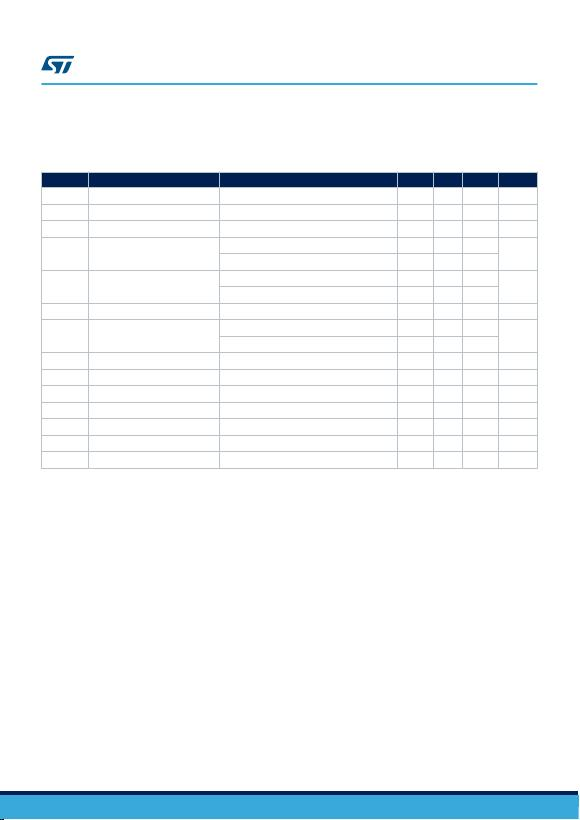Refer to the test circuits, TJ = 0 to 125 °C, VI = 23 V, IO = 500 mA, CI = 0.33 µF, CO = 0.1 µF unless otherwise
specified.
Table 16. Electrical characteristics of L7815C
Symbol Parameter Test conditions Min. Typ. Max. Unit
VOOutput voltage TJ = 25 °C 14.4 15 15.6 V
VOOutput voltage IO = 5 mA to 1 A, VI = 17.5 to 28 V 14.25 15 15.75 V
VOOutput voltage IO = 1 A, VI = 28 to 30 V, TJ = 25 °C 14.25 15 15.75 V
∆VO (1) Line regulation
VI = 17.5 to 30 V, TJ = 25 °C 300
mV
VI = 20 to 26 V, TJ = 25 °C 150
∆VO (1) Load regulation
IO = 5 mA to 1.5 A, TJ = 25 °C 300
mV
IO = 250 to 750 mA, TJ = 25 °C 150
IdQuiescent current TJ = 25 °C 8 mA
∆IdQuiescent current change
IO = 5 mA to 1A 0.5
mA
VI = 17.5 to 30 V 1
∆VO/∆T Output voltage drift IO = 5 mA -1 mV/°C
eN Output noise voltage B = 10 Hz to 100kHz, TJ = 25 °C 90 µV/VO
SVR Supply voltage rejection VI = 18.5 to 28.5 V, f = 120 Hz 54 dB
VdDropout voltage IO = 1 A, TJ = 25 °C 2 V
ROOutput resistance f = 1 kHz 19 mΩ
Isc Short circuit current VI = 35 V, TJ = 25 °C 0.23 A
Iscp Short circuit peak current TJ = 25 °C 2.2 A
1. Load and line regulation are specified at constant junction temperature. Changes in VO due to heating
effects must be taken into account separately. Pulse testing with low duty cycle is used.
Note: Minimum load current for regulation is 5 mA.
L78
Electrical characteristics
DS0422 - Rev 36 page 19/55Refer to the test circuits, TJ = 0 to 125 °C, VI = 26 V, IO = 500 mA, CI = 0.33 µF, CO = 0.1 µF unless otherwise
specified.
Table 17. Electrical characteristics of L7818C
Symbol Parameter Test conditions Min. Typ. Max. Unit
VOOutput voltage TJ = 25 °C 17.3 18 18.7 V
VOOutput voltage IO = 5 mA to 1 A, VI = 21 to 31 V 17.1 18 18.9 V
VOOutput voltage IO = 1 A, VI = 31 to 33 V, TJ = 25 °C 17.1 18 18.9 V
∆VO (1) Line regulation
VI = 21 to 33 V, TJ = 25 °C 360
mV
VI = 24 to 30 V, TJ = 25 °C 180
∆VO (1) Load regulation
IO = 5 mA to 1.5 A, TJ = 25 °C 360
mV
IO = 250 to 750 mA, TJ = 25 °C 180
IdQuiescent current TJ = 25 °C 8 mA
∆IdQuiescent current change
IO = 5 mA to 1 A 0.5
mA
VI = 21 to 33 V 1
∆VO/∆T Output voltage drift IO = 5 mA -1 mV/°C
eN Output noise voltage B = 10 Hz to 100 kHz, TJ = 25 °C 110 µV/VO
SVR Supply voltage rejection VI = 22 to 32 V, f = 120 Hz 53 dB
VdDropout voltage IO = 1 A, TJ = 25 °C 2 V
ROOutput resistance f = 1 kHz 22 mΩ
Isc Short circuit current VI = 35 V, TJ = 25 °C 0.20 A
Iscp Short circuit peak current TJ = 25 °C 2.1 A
1. Load and line regulation are specified at constant junction temperature. Changes in VO due to heating
effects must be taken into account separately. Pulse testing with low duty cycle is used.
Note: Minimum load current for regulation is 5 mA.
L78
Electrical characteristics
DS0422 - Rev 36 page 20/55Refer to the test circuits, TJ = 0 to 125 °C, VI = 33 V, IO = 500 mA, CI = 0.33 µF, CO = 0.1 µF unless otherwise
specified.
Table 18. Electrical characteristics of L7824C
Symbol Parameter Test conditions Min. Typ. Max. Unit
VOOutput voltage TJ = 25 °C 23 24 25 V
VOOutput voltage IO = 5 mA to 1 A, VI = 27 to 37 V 22.8 24 25.2 V
VOOutput voltage IO = 1 A, VI = 37 to 38 V, TJ = 25 °C 22.8 24 25.2 V
∆VO (1) Line regulation
VI = 27 to 38 V, TJ = 25 °C 480
mV
VI = 30 to 36 V, TJ = 25 °C 240
∆VO (1) Load regulation
IO = 5 mA to 1.5 A, TJ = 25 °C 480
mV
IO = 250 to 750 mA, TJ = 25 °C 240
IdQuiescent current TJ = 25 °C 8 mA
∆IdQuiescent current change
IO = 5 mA to 1 A 0.5
mA
VI = 27 to 38 V 1
∆VO/∆T Output voltage drift IO = 5 mA -1.5 mV/°C
eN Output noise voltage B = 10 Hz to 100 kHz, TJ = 25 °C 170 µV/VO
SVR Supply voltage rejection VI = 28 to 38 V, f = 120 Hz 50 dB
VdDropout voltage IO = 1 A, TJ = 25 °C 2 V
ROOutput resistance f = 1 kHz 28 mΩ
Isc Short circuit current VI = 35 V, TJ = 25° C 0.15 A
Iscp Short circuit peak current TJ = 25 °C 2.1 A
1. Load and line regulation are specified at constant junction temperature. Changes in VO due to heating
effects must be taken into account separately. Pulse testing with low duty cycle is used.
Note: Minimum load current for regulation is 5 mA.
L78
Electrical characteristics
DS0422 - Rev 36 page 21/55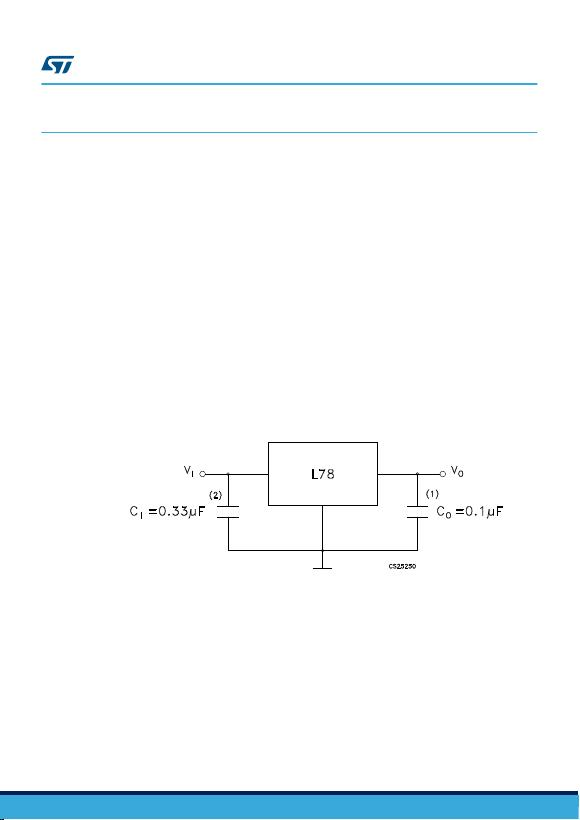6Application information
6.1 Design consideration
The L78 Series of fixed voltage regulators are designed with thermal overload protection that shuts down the
circuit when subjected to an excessive power overload condition, internal short-circuit protection that limits the
maximum current the circuit will pass, and output transistor safe-area compensation that reduces the output short-
circuit current as the voltage across the pass transistor is increased. In many low current applications,
compensation capacitors are not required. However, it is recommended that the regulator input be bypassed with
capacitor if the regulator is connected to the power supply filter with long lengths, or if the output load capacitance
is large. An input bypass capacitor should be selected to provide good high frequency characteristics to insure
stable operation under all load conditions. A 0.33 µF or larger tantalum, mylar or other capacitor having low
internal impedance at high frequencies should be chosen. The bypass capacitor should be mounted with the
shortest possible leads directly across the regulators input terminals. Normally good construction techniques
should be used to minimize ground loops and lead resistance drops since the regulator has no external sense
lead.
The addition of an operational amplifier allows adjustment to higher or intermediate values while retaining
regulation characteristics. The minimum voltage obtained with the arrangement is 2 V greater than the regulator
voltage.
The circuit of Figure 14. High current voltage regulator can be modified to provide supply protection against short
circuit by adding a short circuit sense resistor, RSC, and an additional PNP transistor. The current sensing PNP
must be able to handle the short circuit current of the three terminal regulator Therefore a four ampere plastic
power transistor is specified.
Figure 9. Fixed output regulator
GAMG220920161007MT
1. Although no output capacitor is need for stability, it does improve transient response.
2. Required if regulator is located an appreciable distance from power supply filter.
L78
Application information
DS0422 - Rev 36 page 22/55